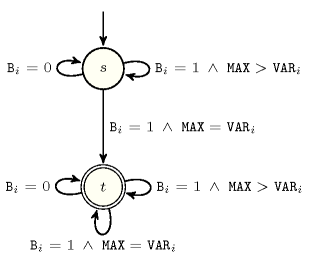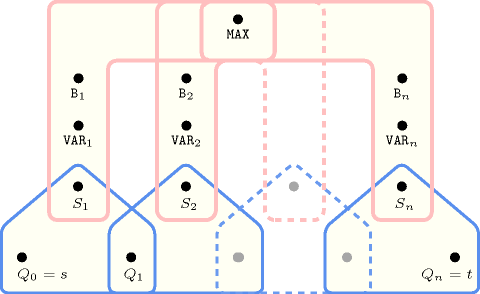## 5.300. open_maximum

Origin
Constraint

$\mathrm{𝚘𝚙𝚎𝚗}_\mathrm{𝚖𝚊𝚡𝚒𝚖𝚞𝚖}\left(\mathrm{𝙼𝙰𝚇},\mathrm{𝚅𝙰𝚁𝙸𝙰𝙱𝙻𝙴𝚂}\right)$

Arguments
 $\mathrm{𝙼𝙰𝚇}$ $\mathrm{𝚍𝚟𝚊𝚛}$ $\mathrm{𝚅𝙰𝚁𝙸𝙰𝙱𝙻𝙴𝚂}$ $\mathrm{𝚌𝚘𝚕𝚕𝚎𝚌𝚝𝚒𝚘𝚗}\left(\mathrm{𝚟𝚊𝚛}-\mathrm{𝚍𝚟𝚊𝚛},\mathrm{𝚋𝚘𝚘𝚕}-\mathrm{𝚍𝚟𝚊𝚛}\right)$
Restrictions
 $|\mathrm{𝚅𝙰𝚁𝙸𝙰𝙱𝙻𝙴𝚂}|>0$ $\mathrm{𝚛𝚎𝚚𝚞𝚒𝚛𝚎𝚍}$$\left(\mathrm{𝚅𝙰𝚁𝙸𝙰𝙱𝙻𝙴𝚂},\left[\mathrm{𝚟𝚊𝚛},\mathrm{𝚋𝚘𝚘𝚕}\right]\right)$ $\mathrm{𝚅𝙰𝚁𝙸𝙰𝙱𝙻𝙴𝚂}.\mathrm{𝚋𝚘𝚘𝚕}\ge 0$ $\mathrm{𝚅𝙰𝚁𝙸𝙰𝙱𝙻𝙴𝚂}.\mathrm{𝚋𝚘𝚘𝚕}\le 1$
Purpose

$\mathrm{𝙼𝙰𝚇}$ is the maximum value of the variables $\mathrm{𝚅𝙰𝚁𝙸𝙰𝙱𝙻𝙴𝚂}\left[i\right].\mathrm{𝚟𝚊𝚛}$, $\left(1\le i\le |\mathrm{𝚅𝙰𝚁𝙸𝙰𝙱𝙻𝙴𝚂}|\right)$ for which $\mathrm{𝚅𝙰𝚁𝙸𝙰𝙱𝙻𝙴𝚂}\left[i\right].\mathrm{𝚋𝚘𝚘𝚕}=1$ (at least one of the Boolean variables is set to 1).

Example
$\left(\begin{array}{c}5,〈\begin{array}{cc}\mathrm{𝚟𝚊𝚛}-3\hfill & \mathrm{𝚋𝚘𝚘𝚕}-1,\hfill \\ \mathrm{𝚟𝚊𝚛}-1\hfill & \mathrm{𝚋𝚘𝚘𝚕}-0,\hfill \\ \mathrm{𝚟𝚊𝚛}-7\hfill & \mathrm{𝚋𝚘𝚘𝚕}-0,\hfill \\ \mathrm{𝚟𝚊𝚛}-5\hfill & \mathrm{𝚋𝚘𝚘𝚕}-1,\hfill \\ \mathrm{𝚟𝚊𝚛}-5\hfill & \mathrm{𝚋𝚘𝚘𝚕}-1\hfill \end{array}〉\hfill \end{array}\right)$

The $\mathrm{𝚘𝚙𝚎𝚗}_\mathrm{𝚖𝚊𝚡𝚒𝚖𝚞𝚖}$ constraint holds since its first argument $\mathrm{𝙼𝙰𝚇}=5$ is set to the maximum value of values $3,1,7,5,5$ for which the corresponding Boolean $1,0,0,1,1$ is set to 1 (i.e., values $3,5,5$).

Typical
 $|\mathrm{𝚅𝙰𝚁𝙸𝙰𝙱𝙻𝙴𝚂}|>1$ $\mathrm{𝚛𝚊𝚗𝚐𝚎}$$\left(\mathrm{𝚅𝙰𝚁𝙸𝙰𝙱𝙻𝙴𝚂}.\mathrm{𝚟𝚊𝚛}\right)>1$
Symmetries
• Items of $\mathrm{𝚅𝙰𝚁𝙸𝙰𝙱𝙻𝙴𝚂}$ are permutable.

• One and the same constant can be added to $\mathrm{𝙼𝙰𝚇}$ as well as to the $\mathrm{𝚟𝚊𝚛}$ attribute of all items of $\mathrm{𝚅𝙰𝚁𝙸𝙰𝙱𝙻𝙴𝚂}$.

Figure 5.300.1 depicts the automaton associated with the $\mathrm{𝚘𝚙𝚎𝚗}_\mathrm{𝚖𝚊𝚡𝚒𝚖𝚞𝚖}$ constraint. Let ${\mathrm{𝚅𝙰𝚁}}_{i},{𝙱}_{i}$ be the ${i}^{th}$ item of the $\mathrm{𝚅𝙰𝚁𝙸𝙰𝙱𝙻𝙴𝚂}$ collection. To each triple $\left(\mathrm{𝙼𝙰𝚇},{\mathrm{𝚅𝙰𝚁}}_{i},{𝙱}_{i}\right)$ corresponds a signature variable ${S}_{i}$ as well as the following signature constraint: $\left({𝙱}_{i}=1\wedge \mathrm{𝙼𝙰𝚇}<{\mathrm{𝚅𝙰𝚁}}_{i}⇔{S}_{i}=0\right)\wedge \left({𝙱}_{i}=1\wedge \mathrm{𝙼𝙰𝚇}={\mathrm{𝚅𝙰𝚁}}_{i}⇔{S}_{i}=1\right)\wedge \left({𝙱}_{i}=1\wedge \mathrm{𝙼𝙰𝚇}>{\mathrm{𝚅𝙰𝚁}}_{i}⇔{S}_{i}=2\right)\wedge \left({𝙱}_{i}=0\wedge \mathrm{𝙼𝙰𝚇}<{\mathrm{𝚅𝙰𝚁}}_{i}⇔{S}_{i}=3\right)\wedge \left({𝙱}_{i}=0\wedge \mathrm{𝙼𝙰𝚇}={\mathrm{𝚅𝙰𝚁}}_{i}⇔{S}_{i}=4\right)\wedge \left({𝙱}_{i}=0\wedge \mathrm{𝙼𝙰𝚇}>{\mathrm{𝚅𝙰𝚁}}_{i}⇔{S}_{i}=5\right)$.
##### Figure 5.300.1. Automaton of the $\mathrm{𝚘𝚙𝚎𝚗}_\mathrm{𝚖𝚊𝚡𝚒𝚖𝚞𝚖}$ constraint##### Figure 5.300.2. Hypergraph of the reformulation corresponding to the automaton of the $\mathrm{𝚘𝚙𝚎𝚗}_\mathrm{𝚖𝚊𝚡𝚒𝚖𝚞𝚖}$ constraint